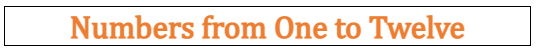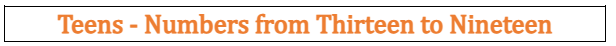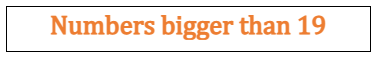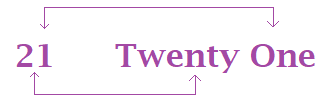# Math > Year 2

## Reading and writing numbers up to 100 in numerals and words0 = Zero

1 = One

2 = Two

3 = Three

4 = Four

5 = Five

6 = Six

7 = Seven

8 = Eight

9 = Nine

10 = Ten13 = Thirteen

14 = Fourteen

15 = Fifteen

16 = Sixteen

17 = Seventeen

18 = Eighteen

19 = NineteenTo read the numbers, we need to look at the first digit and then at the second digit.

For example,72 = Seventy-one20 = Twenty

30 = Thirty

40 = Forty

50 = Fifty

60 = Sixty

70 = Seventy

80 = Eighty

90 = Ninety

100 = Hundred

//